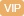| APP | 校园号 | 客服 客服热线：400-863-9889

400-863-9889（2018秋•虎林市校级期末）直接写出得数．
 6.2+3.8= 5-1.6= 1.8×0.5= 0.36÷0.6= 1.43+0.8= 3.9-0.39= 0.48÷0.3= 8.2×0.01=
【考点】小数的加法和减法小数乘法小数除法【专题】计算题．
【分析】根据减法性质进简算；

【解答】解：12
 1 7
-（.5+3
 1 7

=-6.5
=（
 7 9
+
 2 9
）×
 1 6

 7 9
÷6+
 2 9
×
 1 6

=25；
=1×
 1 6

=
 1 6
【点评】考查了运定律与便运算，则混合意运算顺序和运算法则，灵运用所学的运算定律简便计．

0/0